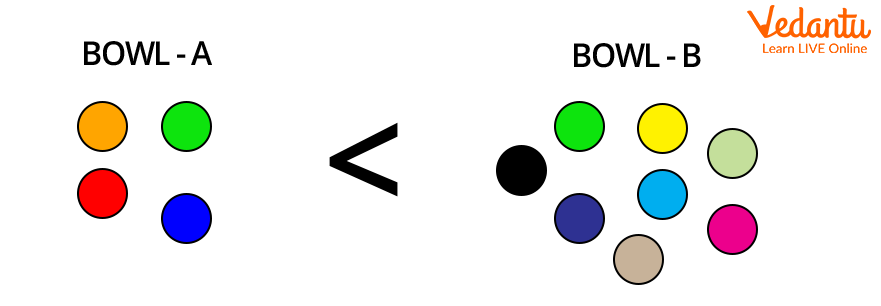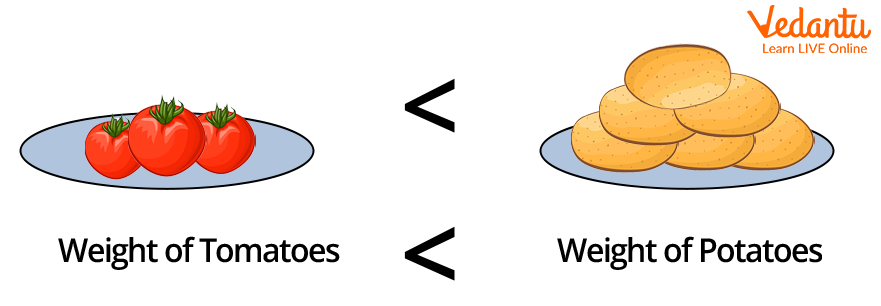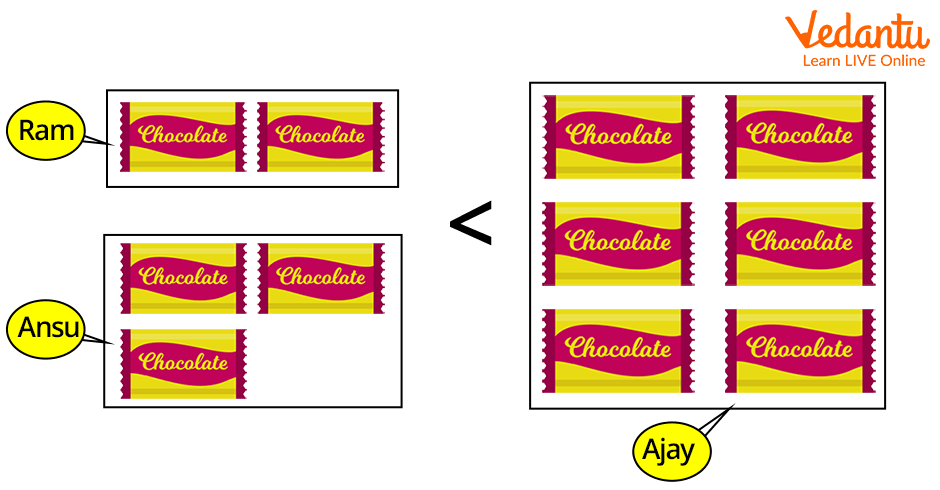Courses
Courses for Kids
Free study material
Free LIVE classes
More

# Less Than: Introduction, Usage and Examples## Introduction to the Less Than Symbol

The less-than symbol is a fundamental mathematical symbol that denotes the difference between two values. In mathematics, there are several symbols that are used to conduct various arithmetic operations, hold some fixed values, and compare numbers using equality and inequality symbols.

In this topic, we'll go through one of the inequality symbols, the "Less than Symbol," in depth, using multiple examples.

## What is a Less than Symbol?

When comparing two values where the first number is less than the second number, such as in ascending order, the less-than symbol is used. The opening angle bracket is represented by the less than symbol, which is an approximation. " " is the symbol used to represent inequality that is less than. The less than sign is a widely used math symbol that consists of two equal-sized strokes that meet at an acute angle on the left.

## Remembering the Less than Sign

The "Alligator Method" is the best way to remember the less-than sign. Consider the alligator approach for comparing two values. The alligator's mouth is considered to constantly point to the highest value because it can consume as much as it wants. The alligator's mouth is always open to the right, symbolising inequality. To employ greater than a symbol, we can use the alligator method. The greater than symbol is defined by the alligator mouth pointing to the left.

## Examples of Less than

One of the words used to show the relationship between two values is less than. It expresses the fact that one value is less than the other. The symbol for less than is, and we can compare numbers, weights, heights, and values using this metric.

For example, in Bowl A, there are four marbles and in Bowl B, there are seven marbles. When the two are compared, it is evident that Bowl A has less marbles than Bowl B.Fig: An example of a less than symbol

Likewise, we can also compare weights. For example:

Ray purchases 4 kg of potatoes and 2 kg of onions and separates them into two bags. His son receives the lighter bag from him. Which one does he cling to?

To do so, you must first determine which bag is lighter. There are two bags, one containing 4 kg of potatoes and the other containing 2 kg of onions. As a result, the onion bag weights less, and Ray gives it to his son while carrying the potato bag.Fig: Finding the weight of vegetables by using the Less than symbol

Ajay has six chocolate packets. Ram has two chocolate packs while Ansu has three chocolate packs. Is Ajay's chocolate collection bigger than Ram's and Ansu's combined?

With Ram and Ansu, the total amount of packets is 2 + 3 = 5 packets.

Ajay also has 6 chocolate packets.Fig: Finding the chocolate box count using the Less than symbol

Therefore, Ram and Ansu together have only fewer chocolates compared to Ajay.

Some more examples of less than with symbol include:

• 0.8 < 1.2: 0.8 is less than 1.2

• 2 < 5: 2 is less than 5

• -0.6 < -0. 1: -0.6 is less than -0.1

## Summary

To summarise, comparing is an important part of our life. It's impossible to demonstrate the equality or inequality between items we see around us without a comparison.

## Fun Fact

Thomas Harriot, a British mathematician, first introduced the symbol for less than () in his book "Artis Analyticae Praxis ad Aequationes Algebraicas Resolvendas" in 1631.

Last updated date: 29th Sep 2023
Total views: 137.7k
Views today: 2.37k

## FAQs on Less Than: Introduction, Usage and Examples

1. What is the use of less than sign

The less-than sign is one of the inequality signs, which is used to compare the number. A less sign is used when the first number is less than the second number. 5 x 10 is a good example. It signifies that 5 is less than 10.

2. What are the other terms used to describe less than?

Smaller than, lower than, below, fewer than, not greater than, and so on are various terms used to describe less than.